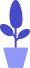# Python function definition

Estimated reading: 3 minutes 52 views

A function is a way of grouping together code that implements some functionality so that it can be reused. Functions allow us to run the same piece of code in multiple places in a program without repeatedly copying and pasting code. Also, by hiding large chunks of code inside functions and giving it an easy-to-understand name, we can better organize and plan our code.

With functions, we can focus on the organization of functions without paying too much attention to all the code details that make up those functions. The smaller and more manageable the code segment is, the more we can see the bigger picture and think about how to build the program at a higher level.

Python has two kinds of functions, one is the built-in function, for example, print(), int() that we have contacted earlier; the other is the custom function that we define and write by ourselves. In this tutorial, we will learn about functions.

## Basic structure of a Python function

In Python, when declaring a custom function, include the following parts:

• def keyword;
• the name of the function;
• parameter list (the number of parameters can be determined as needed);
• colon;
• Indented code starting on the next line;
• The keyword return and the returned result (this part is optional).

Let’s start by creating a simple function.

``````def firstFunction(name):
str1="Hello "+name+"!"
print(str1)``````

First declare that this is a function through the keyword def, and then give the function a name – firstFunction, this function has a parameter called name, we assign the string generated by the statement “Hello “+name+”!” to the variable str1 , then print str1. This function returns no result.

## Arguments of Python functions

If there is a parameter in a function, we call this parameter a formal parameter. The parameter list that each function contains is called the parameter list. The parameters in the parameter list can be one parameter, multiple parameters, or even no parameters. If multiple parameters are used, separate the names of each parameter with commas.

Let’s look at another example, it has two parameters, the code is as follows.

``````def sum(number1,number2):
result=number1+number2
print(str(result))``````

This is a function that performs an addition operation, and its two formal parameters are named number1 and number2. When we call this function, we will pass in two parameters, which we call actual parameters.

sum(12,21)12 and 21 here are the actual parameters. Running this code yields the result shown in Figure 1.